# OliNo

Renewable Energy

## Retail In Motion MR16 5W 2700K 60deg

Posted by Marcel van der Steen in Led lights, Light measurements Add comments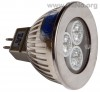presents an MR16 led light bulb. It contains 3 powerleds with each an own specific reflector. This article shows the measurement results. Many parameters are also found in the Eulumdat file.

See this overview for a comparison with other light bulbs.

### Summary measurement data

parameter meas. result remark
Color temperature 2830 K Warm white.
Luminous intensity Iv 105 Cd
Beam angle 69 deg
Power P 3.9 W
Power Factor 0.73 For every 1 kWh net power consumed, there has been 0.9 kVAhr for reactive power.
Luminous flux 112 lm
Luminous efficacy 29 lm/W
CRI_Ra 69 Color Rendering Index.
Coordinates chromaticity diagram x=0.4445 and y=0.3984
Fitting GU5.3/MR16
D x H external dimensions 50 x 40 mm External dimensions of the light bulb. This excludes the pins.
D luminous area 32 mm Dimensions of the luminous area (used in Eulumdat file). It is the diameter of a circle that contains the three reflectors of the leds.
General remarks The ambient temperature during the whole set of measurements was 27-27.5 deg C.
Warm up effect: during the warm up time the illuminance decreases with 20 %.

Voltage dependency: the power consumption and illumination decrease when the voltage drops below 12 V.

Measurement report (PDF)Eulumdat fileRight click on icon and save the file.

### Eulumdat light diagram

An interesting graph is the light diagram, indicating the intensity in the C0-C180 and the C90-C270 plane. This light diagram below comes from the program Qlumedit, that extracts these diagrams from an Eulumdat file.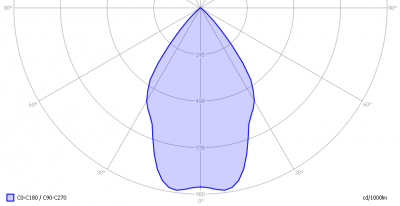The light diagram giving the radiation pattern.

It indicates the luminous intensity around the light bulb. This light bulb has no difference in light diagram between the C0-C180 and C90-C270 plane as the light bulb is symmetrical over its lenth axis (the so called z-axis). C0-C180 and C90-C270 planes are cross sections through the length direction of the light bulb.

The unit is Cd/1000lm, meaning the intensity in Cd assuming there would be 1000 lumen in the measured light bulb. This enables comparing different types of light bulbs.

### Illuminance Ev at 1 m distance, or luminous intensity Iv

Herewith the plot of the averaged luminous intensity Iv as a function of the inclination angle with the light bulb.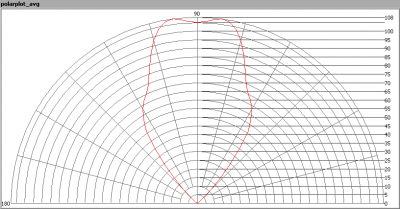The radiation pattern of the light bulb.

This radiation pattern is the average of the light output of the light diagram given earlier. Also, in this graph the luminous intensity is given in Cd.

These averaged values are used (later) to compute the lumen output.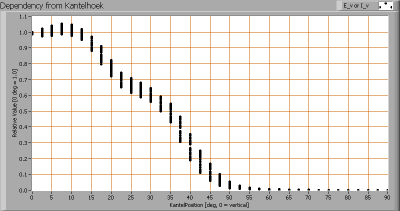Intensity data of every measured turn angle at each inclination angle.

This plot shows per inclination angle the intensity measurement results for each turn angle at that inclination angle. There are little differences in illuminance values for different turn angles. However for further calculations the averaged values will be used.

When using the average values per inclination angle, the beam angle can be computed, being 69 degrees. This is the average value which is valid for any cross section through the z-axis of the light bulb.

### Luminous flux

With the averaged illuminance data at 1 m distance, taken from the graph showing the averaged radiation pattern, it is possible to compute the luminous flux.

The result of this computation for this light spot is a luminous flux of 112 lm.

### Luminous efficacy

The luminous flux being 112 lm, and the power of the lightbulb being 3.9 W, yields a luminous efficacy of 29 lm/W.

A power factor of 0.73 means that for every 1 kWh net power consumed, a reactive component of 0.9 kVAr was needed.

 Light bulb voltage 12.0 VAC Light bulb current 445 mA Power P 3.9 W Apparent power S 5.3 VA Power factor 0.73

Of this light bulb the voltage across ad the resulting current through it are measured and graphed.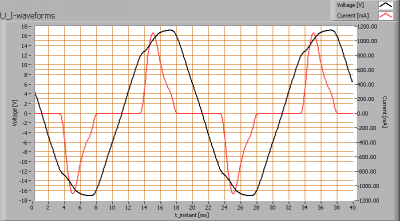Voltage across and current through the lightbulb

The current peaks when the voltage reaches it maximum. There is a big difference in the form between the current and voltage, that decreases the powerfactor.
Also the power spectrum of the current is determined.Current power spectrum in % of the first harmonic (50 Hz).

The current peaks result in a current power spectrum with quite some higher harmonics than the ground harmonic (50 Hz).

### Color temperature and Spectral power distribution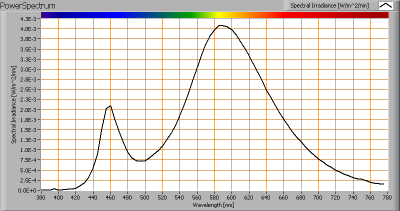The spectral power distribution of this light bulb.

The measured color temperature is about 2850 K which is warm white.

This color temperature is measured straight underneath the light bulb. Below a graph showing the color temperature for different inclination angles.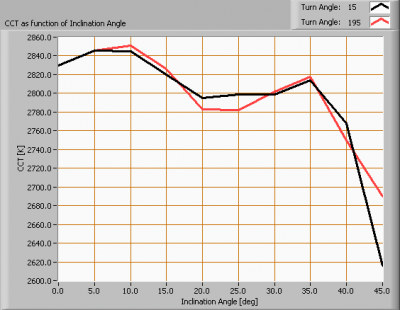Color temperature as a function of inclination angle.

The color temperature is given for inclination angles up to 45 degrees; at higher inclination angles there is little illumination left.

The value remains constant within 6 % of its initial value at 0 degrees inclination angle. When looking until 35 degrees inclination angle, the deviation is within 2 %.

### Chromaticity diagram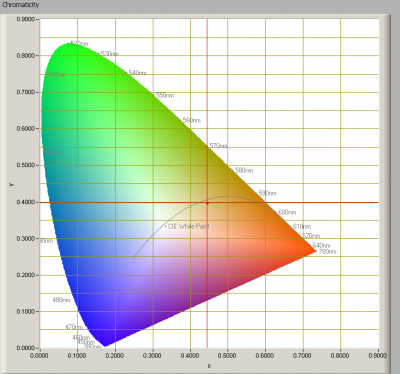The chromaticity space and the position of the lamp’s color coordinates in it.

The light coming from this lamp is somewhat below the Planckian Locus (the black path in the graph).

Its coordinates are x=0.4445 and y=0.3984.

### Color Rendering Index (CRI) or also Ra

Herewith the image showing the CRI as well as how well different colors are represented (rendered). The higher the number, the better the resemblance with the color when a black body radiator would have been used (the sun, or an incandescent lamp).

Each color has an index Rx, and the first 8 indexes (R1 .. R8) are averaged to compute the Ra which is equivalent to the CRI.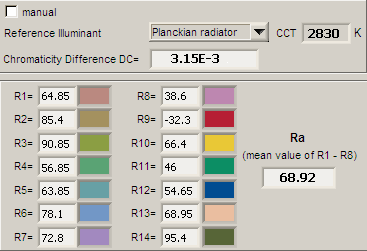CRI of the light of this lightbulb.

The value of 69 is lower than 80 which is considered a minimum value for indoor usage.

Note: the chromaticity difference is 0.0032 indicates the distance to the Planckian Locus. Its value is lower than 0.0054, which means that the calculated CRI result is meaningful.

### Voltage dependency

The dependency of a number of lamp parameters on the lamp voltage is determined. For this, the lamp voltage has been varied and its effect on the following lamp parameters measured: illuminance E_v [lx], the lamppower P [W], the (Correlated) Color Temperature [K] and the luminous efficacy [lm/W].Lamp voltage dependencies of certain light bulb parameters, where the value at 12 V is taken as 100 %.

There is only significant variation of parameters when the voltage drops below the 12 V.

When the voltage at 12 V varies with + and – 0.25 V abruptly (comparable to 5 V on 230 V grid voltage), then the illuminance varies within 2 %, so when abrupt voltage changes occur this effect is not visible in the illuminance output.

### Warm up effects

After switch on of a cold lamp, the effect of heating up of the lamp is measured on illuminance E_v [lx], color temperature CT or correlated color temperature CCT [K], the lamppower P [W] and the luminous efficacy [lm/W].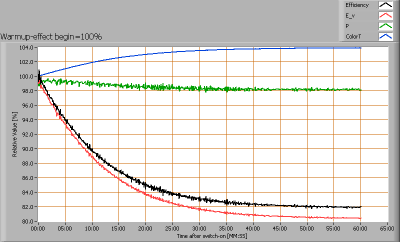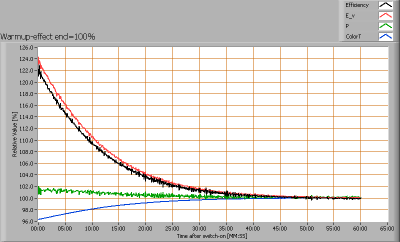Effect of warming up on different light bulb parameters. At top the 100 % level is put at begin, and at bottom at the end.

The warm-up time is about 35 minutes during which the illumination level decreases with 20 %.